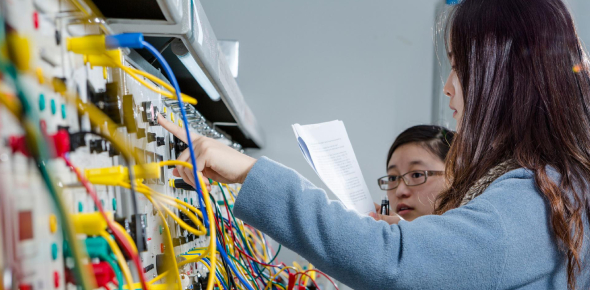# Quiz: Test Your Basic Electricity Knowledge! Trivia

13 Questions | Total Attempts: 53Settings.

• 1.
What is the electrical measurement for resistance?
• A.

Volts

• B.

Amps

• C.

Hertz

• D.

Ohms

• 2.
What is the electrical measurement for current flow?
• A.

Amps

• B.

Ohms

• C.

Volts

• D.

D/C

• 3.
What atomic trait make for a good conductor?
• A.

5 or more electrons on outer ring

• B.

4 electrons on the outer ring

• C.

3 or less electrons on outer ring

• D.

3 or less protons

• 4.
What atomic trait makes for a good insulator?
• A.

5 or more electrons on outer ring

• B.

5 or more protons

• C.

3 or less electrons on outer ring

• D.

3 or less protons

• 5.
What type of circuit has the same voltage to each resistor but different amperage flow?
• A.

Series Parallel circuit

• B.

Simple circuit

• C.

Series circuit

• D.

Parallel circuit

• 6.
Amperage refers to electrical____________.
• A.

Pressure

• B.

Flow

• C.

Electromotive force

• D.

Voltage potential

• 7.
A fully charged automotive battery contains how much voltage?
• A.

12v

• B.

13v

• C.

12.4v

• D.

12.6v

• 8.
What is the amperage in a circuit that has 12v and a 2ohm resistor in ti?
• A.

24amps

• B.

3amps

• C.

6amps

• D.

2amps

• 9.
What is the symbol for A/C voltage?
• A.

Option 1

• B.

Option 2

• C.

Option 3

• D.

Option 4

• 10.
What is the symbol for Diode?
• A.

Option 1

• B.

Option 2

• C.

Option 3

• D.

Option 4

• 11.
What is the symbol for Ohms?
• A.

Option 1

• B.

Option 2

• C.

Option 3

• D.

Option 4

• 12.
What is the function of a diode?
• A.

Allows a/c current to flow

• B.

To block electrical flow in both directions

• C.

Electricity can pass through both directions

• D.

Electricity can only pass through 1 direction

Related TopicsBack to top